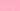Pricing# What is the CAPM formula?

Assessing the relationship between risk and return is at the heart of investment, and analysts have multiple tools in their arsenal to help. One of these is the capital asset pricing model, or CAPM. The CAPM formula is used widely in finance. Here’s how it works.

## What is CAPM?

CAPM stands for capital asset pricing model. It’s a formula used to calculate the expected return on an asset in comparison to its systemic risk. The CAPM formula is used in finance when it comes to pricing risky securities, as well as forecasting expected returns on a given asset given the asset’s risk and cost of capital.

In this case, expected return refers to an asset’s future anticipated profit or loss, based on its known rate of return. Systemic risk refers to the widespread risk inherent in the financial market as a whole, rather than a specific stock or industry. To determine an asset’s expected return against this backdrop of risk, you multiply potential outcomes by their chances of occurrence, totalling the results.

The CAPM simplifies this process for investors with a basic calculation, giving a rough estimate of expected returns vs. risk.

## What does the CAPM formula look like?

The CAPM formula reads as follows:

Expected Return = Risk-free Rate + (Beta x Market Risk Premium)

Here’s a breakdown of what all of these terms mean.

• Expected Return: This is the expected return of any capital asset over time, or a long-term forecast of how the investment will perform.

• Risk-free Rate: The exact rate and time will depend on the country where the investment is made, though it typically refers to the yield on a 10-year government bond.

• Beta: Beta is a measurement of risk, or volatility of returns. It looks at the fluctuation of stock prices in relation to the overall market, or the stock’s market risk sensitivity. For example, if a company’s beta is 1.75, then the stock has 175% volatility in comparison to the market average.

• Market Risk Premium: Market Risk Premium is calculated by subtracting the risk-free rate from the expected market return. It represents any expected returns above the risk-free rate. The more volatile the market is, the higher this figure will be.

## How is the CAPM model used?

When making riskier investments, investors expect to receive higher compensation. In the CAPM formula, the risk-free rate represents the time value of money, since it’s a long-term yield. The other parts of the CAPM model listed above account for the additional risk that the investor takes on.

The beta figure is particularly important when understanding CAPM, measuring the risk an investment adds to a portfolio. When the beta is higher than one, the stock is riskier than the market average. If it’s lower than one, the stock’s risk reduces a portfolio’s risk. Multiplying the beta by the market risk premium and adding the risk-free rate gives the investor a bigger picture of the required return that they need to determine an asset’s value. With this information in hand, investors can balance risk with return to determine whether the stock’s worth their time and money.

## Benefits and limitations of CAPM

There are a few benefits to using the CAPM model, including the fact that it’s simple to use and understand. It offers a straightforward way to account for systematic risk in the stock market, allowing investors to diversify their portfolios accordingly.

However, there are also limitations to this model as certain CAPM assumptions must be made for it to hold true. Limitations of CAPM could include:

• Past returns are calculated that might not most accurately reflect future returns

• It doesn’t cover all investment risks

• CAPM assumptions include the ability to borrow and lend at risk-free rates

• Risk-free rates don’t always remain constant

• It doesn’t evaluate all reasonable returns

While the CAPM model is certainly a useful tool for investors, it might be overly simplistic. It’s best to take in a range of models before making any final investment decisions.

## We can help

GoCardless helps you automate payment collection, cutting down on the amount of admin your team needs to deal with when chasing invoices. Find out how GoCardless can help you with ad hoc payments or recurring payments.

GoCardless makes it easy to collect recurring payments